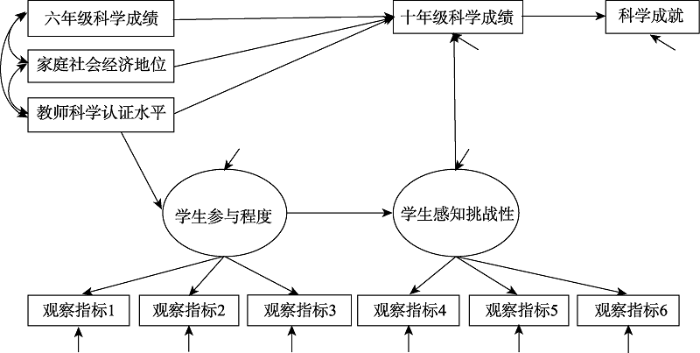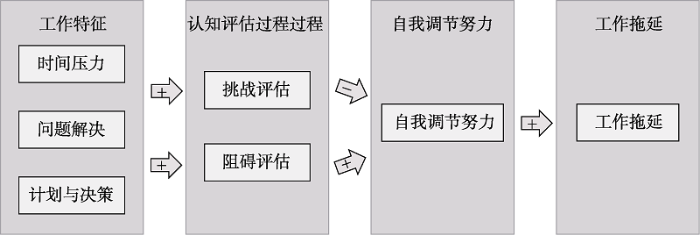ISSN 1671-3710
CN 11-4766/R

## Bayesian structural equation modeling and its current researches

ZHANG Lijin, LU Jiaqi, WEI Xiayan, PAN Junhao,

Department of Psychology, Sun Yat-sen University, Guangzhou 510006, China

 基金资助: * 国家自然科学基金项目.  31871128教育部人文社会科学研究规划基金项目.  18YJA190013中山大学2018年大学生创新训练计划项目.  201810558015Abstract

Structural equation modeling (SEM) has been widely used in psychological researches to investigate the casual relationship among latent variables. Model estimation can be conducted under both the frequentist framework (e.g., maximum-likelihood approach) and the Bayesian framework. In recent years, with the prevalence of Bayesian statistics and its advantages in dealing with small samples, missing data and complex models in SEM, Bayesian structural equation modeling (BSEM) has developed rapidly. However, in China its application in the field of psychology is still insufficient. Therefore, this paper mainly focuses on presenting this new research method to applied researchers. We explain the theoretical and methodological basis of BSEM, as well as its advantages and disadvantages compared with the traditional frequentist approach. We also introduce several commonly used BSEM models and their applications.

Keywords： structural equation modeling ; Bayesian estimation ; maximum-likelihood estimation

ZHANG Lijin, LU Jiaqi, WEI Xiayan, PAN Junhao. Bayesian structural equation modeling and its current researches. Advances in Psychological Science[J], 2019, 27(11): 1812-1825 doi:10.3724/SP.J.1042.2019.01812

## 1 引言

$${{y}_{i}}=\mu +\mathbf{\Lambda }{{\omega }_{i}}+{{\varepsilon }_{i}}$$, i=1, 2, …, n

$${{\eta }_{i}}=\mathbf{\Pi }{{\eta }_{i}}+\mathbf{\Gamma }{{\xi }_{i}}+{{\delta }_{i}}={{\mathbf{\Lambda }}_{\omega }}{{\omega }_{i}}+{{\delta }_{i}}$$

## 2 贝叶斯结构方程模型

(1)设定模型并为未知参数提供先验信息：令k = 1, …, p, h = 1, …, q1, 对于SEM中不同的未知参数提供如下所示的共轭先验分布(李锡钦, 2011)：

${{\mathbf{\Lambda }}_{k}}\tilde{\ }N\left( {{\mathbf{\Lambda }}_{0k}},\ {{\mathbf{H}}_{0k}} \right)$, ${{\mathbf{\Lambda }}_{\omega h}}\tilde{\ }$ $N\left( {{\mathbf{\Lambda }}_{0\omega h}},\ {{\mathbf{H}}_{0\omega h}} \right)$, ${{\text{ }\!\!\Phi\!\!\text{ }}^{-\text{1}}}\text{ }\!\!\tilde{\ }\!\!\text{ Wishart(}{{\text{R}}_{\text{0}}}\text{,}{{\rho }_{\text{0}}}\text{)}$ $\mu \tilde{\ }N\left( {{\mu }_{0}},~{{\mathbf{H}}_{\mu 0}} \right)$

(2)设定MCMC算法迭代次数, 在其收敛后进行模型拟合评估和参数估计。算法是否达到收敛可以通过踪迹图和潜在尺度缩减因子进行评估(详见王孟成 等, 2017);

(3)模型与数据的整体拟合程度可以通过后验预测检验(Posterior Predictive Checking)评估;

(4)为避免先验信息主观性的影响, 研究者可以通过敏感性分析(Sensitivity Analysis; Greenland, 2001)检验不同先验信息下估计结果是否稳定, 增强结果的可靠性。

## 3 分类

### 3.1 经典贝叶斯结构方程模型

3.1.1 贝叶斯验证性因子分析

Muthén和Asparouhov (2012)创新地提出了一种结合了探索和验证方法的贝叶斯验证性因子分析模型, 放宽了模型对于测量误差相关或交叉载荷的限制。在传统方法中, 交叉载荷和测量误差间的相关被严格限制为0, 这既是基于模型简洁性的考虑, 也是因为如果自由估计这些参数容易导致模型不可识别。但是Muthén和Asparouhov (2012)提出的方法在保证模型可识别的同时, 可以通过对交叉载荷提供一个均值为0、方差极小的正态先验分布, 或对误差项矩阵提供合适的逆Wishart分布来放宽对其的限制。模拟研究显示, 该方法在放宽对于交叉载荷或测量误差相关的限制时, 得到的显著的交叉载荷或测量误差相关的数目比修正指数方法得到的更少, 且模型拟合在一次分析中就可以得到满意的结果, 而传统方法通常需要进行多次修正。

Lu等人(2016)指出Muthén和Asparouhov (2012)的方法本质上是将贝叶斯Ridge正则化(Regularization)方法应用于CFA模型中。针对其存在的上述问题, Lu等人(2016)引入了另一种贝叶斯正则化方法：通过对载荷矩阵提供spike-and- slab先验分布, 保留重要的交叉载荷, 将其它微弱的交叉载荷压缩到零。这种方法避免了Ridge正则化方法可能导致的模型过拟合, 及其对重要交叉载荷的过度压缩等问题。

Pan等人(2017)则针对误差项的方差协方差矩阵, 将协方差Lasso (Least absolute shrinkage and selection operator)正则化方法引入CFA模型。通过估计稀疏化的误差协方差矩阵, 在放宽对测量误差相关限制的同时, 将微弱的、不重要的测量误差相关向零压缩, 避免因为测量误差相关过多而导致的模型过拟合或误差项矩阵不正定等问题。其实证研究发现在允许“少量”测量误差相关的情况下, CFA模型的简约性和拟合程度都得到了满足。

3.1.2 贝叶斯结构方程模型

### 图13.1.3 贝叶斯中介模型

### 3.2 贝叶斯潜变量增长曲线模型

LGCM适用于在几个固定时间点观测得到的纵向研究数据(刘红云, 孟庆茂, 2003)。一个简单的非条件线性潜变量增长曲线模型的定义如下(王济川, 王小倩, 姜宝法, 2011)：

$${{y}_{ti}}={{\eta }_{0i}}+{{\lambda }_{t}}{{\eta }_{1i}}+\text{ }\!\!~\!\!\text{ }{{\mathbf{\varepsilon }}_{ti}}$$
$${{\eta }_{0i}}={{\eta }_{0}}+{{\zeta }_{0i}}$$
$${{\eta }_{1i}}={{\eta }_{1}}+{{\zeta }_{1i}}$$

### 图2### 3.3 贝叶斯多组结构方程模型

de Bondt和van Petegem (2015)在该方法的基础上, 基于Asparouhov和Muthén (2014)的建议, 通过对参数跨组的差异值提供先验分布来放宽对于参数严格跨组不变的限制, 检验过度激动问卷的性别跨组不变性。发现在传统方法中模型拟合被拒绝的多组模型, 在放宽了对参数的严格限制后, 拟合良好, 满足了测量不变性。我们将以该研究为例, 详细介绍贝叶斯多组结构方程建模的具体实现步骤。

de Bondt和van Petegem (2015)采用贝叶斯多组结构方程模型来检验过度激动问卷的性别跨组不变性。研究收集了516名大学生的数据, 要求被试在网上填写过度激动问卷, 其中问卷包括精神运动、感官、智力、想象和情感5个维度, 共50题。

### 图3## 5 软件介绍

(1) 目前最为流行的潜变量分析软件Mplus (Muthén & Muthén, 1998~2019),其编程语言简单易学,由于其默认设定较多,在使用中研究者一般只需要将估计方法从极大似然估计换为贝叶斯估计,并提供相应先验信息即可。如果没有提供先验信息Mplus通常会默认提供无信息先验分布。此外, Mplus软件的更新速度很快, Mplus 8.3版本已经可以估计贝叶斯潜调节模型(即调节变量为潜变量的模型)、贝叶斯渐近测量不变性模型等。而当研究者将估计方法设定为贝叶斯估计时, Mplus 8.3可以提供PPp值、DIC和BIC值作为模型拟合与评价的指标;

(2) WinBUGS (Windows version of Bayesian Inference Using Gibbs Sampling; Lunn et al., 2000)是专门用于贝叶斯统计推断的软件包。相比于Mplus, 其对研究者的贝叶斯统计基础有更高的要求。Mplus中一些默认的估计设定在WinBUGS中需要研究者自己设定, 但是它功能强大, 可以灵活地对复杂模型进行估计。WinBUGS能提供DIC值作为模型拟合与评价的指标;

(3) 软件Stan (Stan Development Team, 2014)可以对复杂的BSEM(如潜调节模型、多层模型等)进行估计, 且可以与最流行的数据分析语言(如R, Matlab, Python等)接口;

(4) R软件中的blavaan包(Merkle, & Rosseel, 2015)尚不能估计一些特殊的模型, 如潜调节模型、含有序分类变量的模型等。不过用户可以根据需要导出JAGS (Just Another Gibbs Sampler; Plummer, 2005)代码来估计复杂、特殊的模型。此外, 通过mplus2lavaan()函数还可以将Mplus软件与blavaan接口。blavaan能提供PPp值以及DIC值作为模型拟合与评价的指标。

## 6 讨论

/

 〈〉